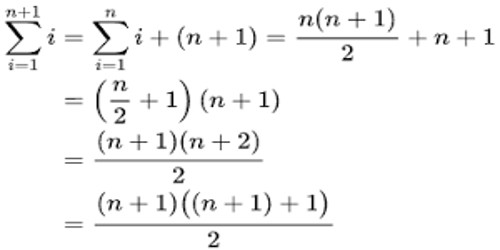Mathematic

# Induction Proof – Problems with SolutionsInduction Proof – Problems with Solutions

Mathematical induction is a technique for proving a statement – a theorem, or a formula — that is asserted about every natural number. It is a mathematical proof technique used to prove a given statement about any well-ordered set. Most commonly, it is used to establish statements for the set of all natural numbers.

1 + 2 + 3 + 4 + ..… + n = {n(n + 1)}/2.

For all positive integers n.

We denote this mathematical statement by P(n). If n = 1, then we find that {1(1 + 1)}/2= 1.

Hence the above statement is true for n = 1.

Suppose n = 2. Then 1 + 2 = 3 and {2(2 + 1)}/2= 3. So we find that the statement is true for n = 2.

Thus, it is easy to verify that P(n) is true for n = 1, 2, 3 or 4. But it is impossible to verify that the above statement is true for all positive integers.

In math induction proof we will work on some examples using mathematical induction.

Mathematical Induction – Problems with Solutions (induction proof):

1. Using the principle of mathematical induction, prove that n(n + 1)(n + 5) is a multiple of 3 for all n N.

Solution:

Let P(n): n(n + 1)(n + 5) is a multiple of 3.

For n = 1, the given expression becomes (1 × 2 × 6) = 12, which is a multiple of 3.

So, the given statement is true for n = 1, i.e. P(1) is true.

Let P(k) be true. Then,

P(k): k(k + 1)(k + 5) is a multiple of 3

⇒ K(k + 1)(k + 5) = 3m for some natural number m, … (i)

Now, (k + 1l)(k + 2)(k + 6) = (k + 1)(k + 2)k + 6(k + 1)(k + 2)

= k(k + 1)(k + 2) + 6(k + 1)(k + 2)

= k(k + 1)(k + 5 – 3) + 6(k + 1)(k + 2)

= k(k + 1)(k + 5) – 3k(k + 1) + 6(k + 1)(k + 2)

= k(k + 1)(k + 5) + 3(k + 1)(k +4) [on simplification]

= 3m + 3(k + 1 )(k + 4) [using (i)]

= 3[m + (k + 1)(k + 4)], which is a multiple of 3

⇒ P(k + 1): (k + 1 )(k + 2)(k + 6) is a multiple of 3

⇒ P(k + 1) is true, whenever P(k) is true.

Thus, P(1) is true and P(k + 1) is true, whenever P(k) is true.

Hence, by the principle of mathematical induction, P(n) is true for all n ∈ N.

1. Using the principle of mathematical induction, prove that (n² + n) is even for all n N.

Solution:

Let P(n): (n² + n) is even.

For n = 1, the given expression becomes (1² + 1) = 2, which is even.

So, the given statement is true for n = 1, i.e., P(1)is true.

Let P(k) be true. Then,

P(k): (k² + k) is even

⇒ (k² + k) = 2m for some natural number m. … (i)

Now, (k + 1)² + (k + 1) = k² + 3k + 2

= (k² + k) + 2(k + 1)

= 2m + 2(k + 1) [using (i)]

= 2[m + (k + 1)], which is clearly even.

Therefore, P(k + 1): (k + 1)² + (k + 1) is even

⇒ P(k + 1) is true, whenever P(k) is true.

Thus, P(1) is true and P(k + 1) is true, whenever P(k) is true.

Hence, by the principle of mathematical induction, P(n)is true for all n ∈ N.

Information Source: